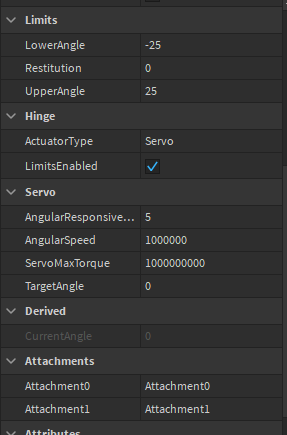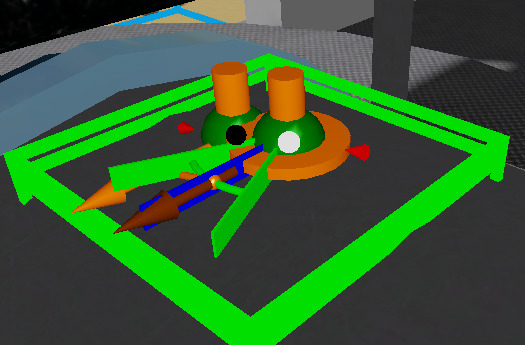# Hingeconstraint only limits correctly facing one way

i made a turret that follow their mouse and moves to there but it only moves correctly when facing one way

hinge:positions:video:
what is it doing:
https://streamable.com/tbkali
what is it suppose to do:
https://streamable.com/qumru9

1 Like

Please post your code so we can see what’s going on ^.^

this is what moves it i forgot to post it

``````mouse.Move:Connect(function()
local delta = mouse.Hit.Position - gun.gun.base.CFrame.Position

gun.gun.base.HingeConstraint.TargetAngle = math.deg(math.atan2(delta.Z, delta.X))
end)``````

Since it’s going backwards, try making the target angle be `-math.deg(math.atan2(delta.Z, delta.X))`

nothing changed at all its still the same

fixed it changed it to

`gun.gun.base.HingeConstraint.TargetAngle = math.deg(math.atan2(-delta.Z, -delta.X))`